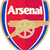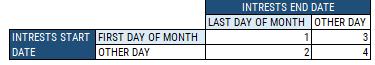# QlikView App Dev

Discussion Board for collaboration related to QlikView App Development.

Announcements
Support Cases coming to Qlik Community Oct. 4! Start chats, open cases, explore resources: READ DETAILS
cancel
Showing results for
Did you mean:Creator

## Interest rates

Hello Experts!
I have interesting task to solve. I’m trying to calculate the interest value and I have a problem to set the correct value of interest rate.

I have two tables
1. Deposits
a. ID
b. VALUE
c. START_DATE
d. END_DATE

2. Interest rate
a. DATE_FROM
b. INTREST_RATE

I’ve transformed those tables, add calendar, add reference table and finally I have got data model as attached.

The interest value are calculated monthly – so I add the list box with period (always one selected value).
The problem I have Is that the interest rate that should be used depends on deposit_start_date and deposit_end_date. Only one interest rate should be used for one deposit in specific month. The rule of determining the interest rate (model) is this:MODEL INTREST RATE 1 LAST DAY OF MONTH 2 START DAY 3 END DAY 4 END DAY

Example:
Interest value for 202003

 ID START_DATE END_DATE END_DATE_MOD DEP_AMOUNT INTEREST START DATE INTEREST END DATE INTEREST MODEL INTEREST RATE DATE INTEREST RATE VALUE 25 03.01.2020 - 01.09.2020 17 090,92 01.03.2020 31.03.2020 1 31.03.2020 1,17% 21 21.03.2020 - 01.09.2020 11 222,52 21.03.2020 31.03.2020 2 21.03.2020 1,18% 2 14.02.2020 03.03.2020 03.03.2020 12 729,68 01.03.2020 02.03.2020 3 02.03.2020 1,71% 42 03.03.2020 12.03.2020 12.03.2020 12 477,58 03.03.2020 11.03.2020 4 11.03.2020 1,69%

Anny suggestions how should I arrange that?

Regards,

Labels (2)

• ### Set Analisys

1 Solution

Accepted SolutionsMVP

May be use a different expression for each model....

``````Pick([INTEREST MODEL],
Avg(Aggr(If(DATE_REF = Max(TOTAL <ID> DATE_REF), IR.IR), ID, DATE_REF)),
Avg(Aggr(If(DATE_REF = Min(TOTAL <ID> DATE_REF), IR.IR), ID, DATE_REF)),
Avg(Aggr(If(DATE_REF = Max(TOTAL <ID> DATE_REF), IR.IR), ID, DATE_REF)),
Avg(Aggr(If(DATE_REF = Max(TOTAL <ID> DATE_REF), IR.IR), ID, DATE_REF)))``````

If only Model 2 has a different expression, you can use an if statement like this

``````If([INTEREST MODEL] = 2,
Avg(Aggr(If(DATE_REF = Min(TOTAL <ID> DATE_REF), IR.IR), ID, DATE_REF)),
Avg(Aggr(If(DATE_REF = Max(TOTAL <ID> DATE_REF), IR.IR), ID, DATE_REF)))``````
5 RepliesCreator
Author

@sunny_talwar  probably You are my last resort. Could You take a look at this?MVP

I was able to get the interest rates to show up, but I am confused as to why we are only looking to get 4 rows? why ID = 5 not show in your output? I am not sure why is that excluded?Creator
Author

Hello @sunny_talwar  those 4 records were only the examples 🙂

I feel we are close but for example for ID 21 the formula shows wrong value, in this case interest rate should be taken from start date or min(date_ref)MVP

May be use a different expression for each model....

``````Pick([INTEREST MODEL],
Avg(Aggr(If(DATE_REF = Max(TOTAL <ID> DATE_REF), IR.IR), ID, DATE_REF)),
Avg(Aggr(If(DATE_REF = Min(TOTAL <ID> DATE_REF), IR.IR), ID, DATE_REF)),
Avg(Aggr(If(DATE_REF = Max(TOTAL <ID> DATE_REF), IR.IR), ID, DATE_REF)),
Avg(Aggr(If(DATE_REF = Max(TOTAL <ID> DATE_REF), IR.IR), ID, DATE_REF)))``````

If only Model 2 has a different expression, you can use an if statement like this

``````If([INTEREST MODEL] = 2,
Avg(Aggr(If(DATE_REF = Min(TOTAL <ID> DATE_REF), IR.IR), ID, DATE_REF)),
Avg(Aggr(If(DATE_REF = Max(TOTAL <ID> DATE_REF), IR.IR), ID, DATE_REF)))``````Creator
Author

@sunny_talwar  You are my Hero! I owe you a good beer - let me know if you'll ever be in Poland. Big Thanks!Community Browser